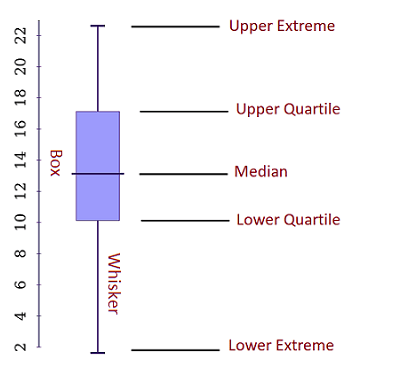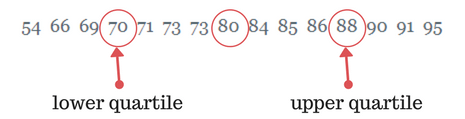# Box and Whisker Plot Examples

When it comes to visualizing a summary of a large data in 5 numbers, many real-world box and whisker plot examples can show you how to solve box plots.

As many other graphs and diagrams in statistics, box and whisker plot is widely used for solving data problems. Believe it or not,  interpreting and reading box plots can be a piece of cake.

On this page:

• What is box and whisker plot? Definition, explanation, and analysis.
• Easy real-life example: problems with answers and interpretation.
• Comparative double box and whisker plot example: to see how to compare two data sets with analysis and interpretation.

Let’s define it:

A box and whisker plot (also known as a box plot) is a graph that represents visually data from a five-number summary. These numbers are median, upper and lower quartile, minimum and maximum data value (extremes).

Don’t panic, these numbers are easy to understand. Look at the following example of box and whisker plot:So, there are a couple of things, you should know in order to work with box plots:

• Lower Extreme – the smallest value in a given dataset.
• Upper Extreme – the highest value in a given dataset.
• Median value – the middle number in the set.
• Lower Quartile – below that value, the lower 25% of the data are contained.
• Upper Quartile – above that value, the upper 25% of the data are contained.
• “Whiskers” – the lines that extend from the boxes. They are used to indicate variability out of the upper and lower quartiles.

To put it in another way, we have 3 key points. The first is the middle point (the median). The others are the middle points of the two halves. These three points divide the data set into quarters, that we call “quartiles”.

We will make the things clearer with a simple real-world example. It also illustrates the steps for solving a box and whisker plot problem.

Example 1: a simple box and whisker plot

Suppose you have the math test results for a class of 15 students. Here are the results:

91  95  54  69  80  85  88  73  71  70  66  90  86  84  73

It is hard to say what is the middle point (the median) because the value points are not ordered.

Step 1: Order the data points from least to greatest.

54  66  69  70  71  73  73  80  84  85  86  88  90  91  95

Step 2: Find the median of the data:This is an odd set of data – you have 15 data points. It means the middle point is 80 as there are 7 data points above it and 7 numbers below.

More on how to calculate median, you can see on our post descriptive statistics examples.

Step 3: Find the middle points of the two halves divided by the median (find the upper and lower quartiles).Step 4: Find the extreme values.

This is the easiest part. You need to find the largest and smallest data values.

Extreme values = 54 and 95.

So, we can determine that the five-number summary for the class of students is 54, 70, 80, 88, 95.

Now we are absolutely ready to draw our box and whisker plot.As you see, the plot is divided into four groups: a lower whisker, a lower box half, an upper box half, and an upper whisker. Each of those groups shows 25% of the data because we have an equal amount of data in each group.

Interpreting the box and whisker plot results:

The box and whisker plot shows that 50% of the students have scores between 70 and 88 points.

In addition, 75% scored lower than 88 points, and 50% have test results above 80. So, if you have test results somewhere in the lower whisker, you may need to study more.

It was among the simplest box and whisker plot examples just to illustrate what the plot shows. Let’s deep further and see double box and whisker plot examples that help you to compare 2 data sets.

Example 2comparative double box and whisker plot

Suppose an IT company has two stores that sell computers. The company recorded the number of sales each store made each month. In the past 12 months, we have the following numbers of sold computers:

Store 1:

350, 460, 20, 160, 580, 250, 210, 120, 200, 510, 290, 380.

Store 2:

520, 180, 260, 380, 80, 500, 630, 420, 210, 70, 440, 140.

In order to compare the two stores sales performance, we will make two box and whisker plots, one for Store 1 and one for Store 2.

Store 1:

First, we put the data points in ascending order.

20, 120, 160, 200, 210, 250, 290, 350, 380, 460, 510 580.

Now, we need to find the median. However, this is an even set of data. There isn’t only one middle point. The middle in our case belongs to sixth + seventh data points e.g. 250 and 290.

And the formula for the median in an even data set is:

(the sum of the two middle numbers) / 2

The median is (250 + 290) / 2 = 270

Now let’s see what happens with the lower and upper quartiles in an even data set:

There are six numbers below the median, namely: 20, 120, 160, 200, 210, 250.

Lower quartile is the median of these six items, so
= (third + fourth data point) / 2
= (160 + 200) / 2
= 180

There are also six numbers above the median, namely: 290, 350, 380, 460, 510 580.

Upper quartile is the median of these six data points.
= (third + fourth data points) / 2
= 420

Finally, the five-number summary for Store 1’s sales is 20, 180, 270, 420, 580.

Using the same calculations, we can find that the five-number summary for Store 2 is 70, 160, 320, 470, 630

Now, we are ready to draw our comparative double box and whisker plot example:Interpreting the results:

Store 2’s highest and lowest sales are both higher than Store 1’s relevant sales.

In addition, Store 2’s median sales value is higher than Store 1’s. Also, Store 2’s interquartile range is larger.

These results tell us that Store 2 consistently sells more computers than Store 1.

How to create box and whisker plots?

Nowadays, you have a plenty of good choices. Depending on your needs, you can create them with a free graphing software such as:

• Plot.ly
• Meta-chart.com

Or you can use powerful premium software such as:

Of course, you can draw them with Microsoft products such as:

• Word
• Excel
• PowerPoint

Finally, just use a sheet of paper or a whiteboard to draw your box plot.

Conclusion

The above box and whisker plot examples aim to help you understand better how to solve them.

Box plots are among the most used types of graphs in the business, statistics and data analysis.

It is especially useful when you want to see if a distribution is skewed and whether there are potential unusual data values (outliers) in a given dataset.

These plots are also widely used for comparing two data sets.

A box plot is a great way of summarizing data set measured on an interval scale (see interval data examples).

It has many advantages and benefits and the most important of them are:

• Able to handle and present a large amount of data.
• A visually effective method of viewing a summary.
• A graphical way showing outliers.
• Great for comparison of two or more datasets.

Need more graph examples? Check our post about simple linear regression examples and Venn diagram examples.